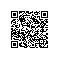# 在java中使用循环定义会出现哪些问题？

public class
ClassX{
public static final int= 2*

ClassY.Y;
}

public class

ClassY{
public static final int=ClassZ.Z+ 1

;
}

public class  ClassZ  extends
ClassX{
public static final int Z= X + 3

;
}

public class
ClassTest{
public static void

main(String[] args){
System.out.println(ClassX.X
+ ClassY.Y +
ClassZ.Z);
}public class

ClassTest{
public staticvoid

main(String[] args){
System.out.println(ClassZ.Z
+ ClassY.Y +
ClassX.X);
}
}1.   X = 2 * ClassY.Y;      计算X，需要知道ClassY.Y的值，下一步计算Y值
2.   Y = ClassZ.Z + 1;      计算Y，需要知道ClassZ.Z的值，下一步计算Z值
3.   Z = X + 3;                计算Z，需要知道X的值，而此时X的值还没有被计算出来(又转回来了，居然是个圈，呵呵^+^)，所以我们使用X的默认值0。

Z = 3;
Y = 4;
X = 8;

1. Z = X + 3;
2. X = 2 * ClassY.Y;
3. Y = ClassZ.Z + 1;(此时使用Z的默认值0)

Y = 1;
X = 2;
Z = 5;使用钉钉扫一扫加入圈子
+ 订阅